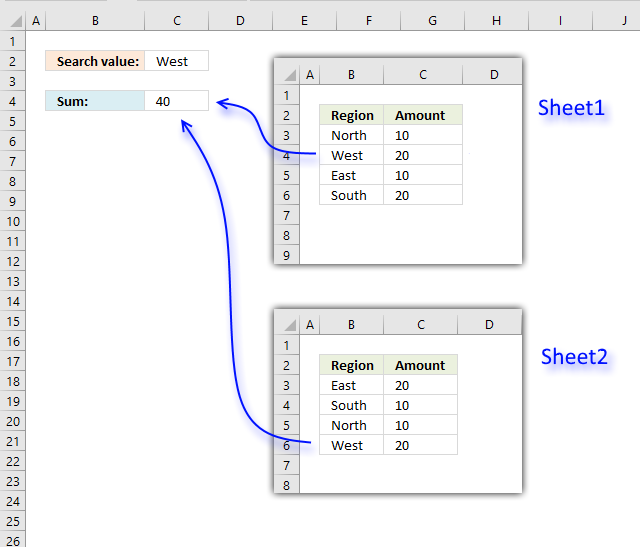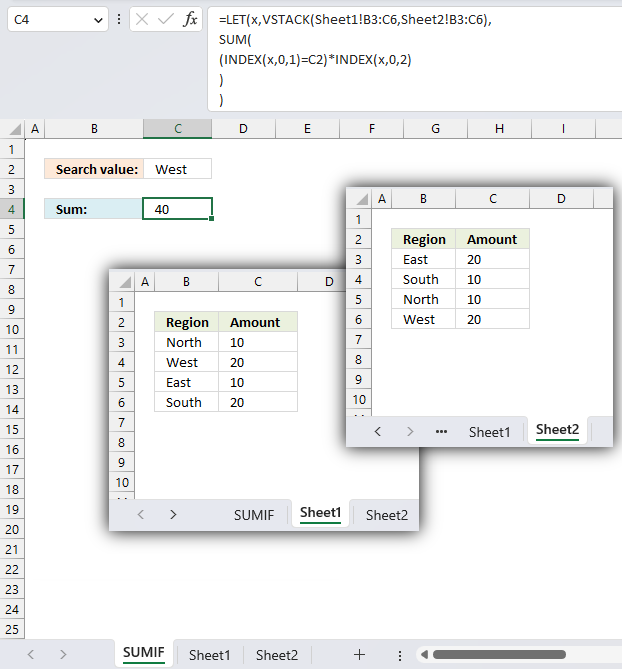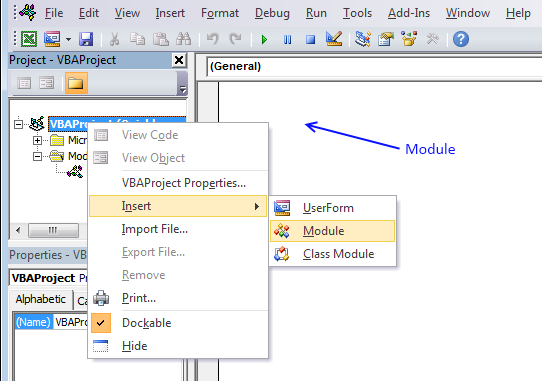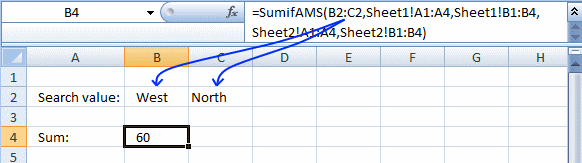Author: Oscar Cronquist Article last updated on March 29, 2023This post describes two ways to a search multiple ranges and calculate a total of the corresponding values across worksheets in a workbook. One is an Excel 365 formula and the second is a User Defined Function.

## 1. SUMIF across multiple sheets - Excel 365 formulaThe image above shows three different worksheets: SUMIF, Sheet1, and Sheet2. The formula in cell C4 adds the amounts if the corresponding value on the same rows are equal to the specified condition in cell C2.

The example above has one match in Sheet1 on row 4 and one match in Sheet2 on row 6. They are 20 and 20 and the total is 40. The formula returns 40 in cell C4.

The SUMIF function is not going to accept an array (only cell range) in the first argument, we need to find a workaround. The SUM function works just as fine if we create a simple logical expression. See the below explanation for more details.

Excel 365 formula in cell C4:

=LET(x,VSTACK(Sheet1!B3:C6,Sheet2!B3:C6),
SUM(
(INDEX(x,0,1)=C2)*INDEX(x,0,2)
)
)

### Explaining formula

#### Step 1 - Stack arrays vertically

The VSTACK function combines cell ranges or arrays. Joins data to the first blank cell at the bottom of a cell range or array (vertical stacking)

Function syntax: VSTACK(array1,[array2],...)

VSTACK(Sheet1!B3:C6,Sheet2!B3:C6)

becomes

VSTACK(
{"North",10;
"West",20;
"East",10;
"South",20},
{"East",20;
"South",10;
"North",10;
"West",20})

and returns

{"North",10;
"West",20;
"East",10;
"South",20;
"East",20;
"South",10;
"North",10;
"West",20})

#### Step 2 - Get the first column from the stacked array

The INDEX function returns a value or reference from a cell range or array, you specify which value based on a row and column number.

Function syntax: INDEX(array, [row_num], [column_num])

INDEX(VSTACK(Sheet1!B3:C6,Sheet2!B3:C6),0,1)

becomes

INDEX({"North",10;
"West",20;
"East",10;
"South",20;
"East",20;
"South",10;
"North",10;
"West",20}),0,1)

and returns

{"North";
"West";
"East";
"South";
"East";
"South";
"North";
"West"})

#### Step 3 - Create logical expression

The equal sign is a logical operator, it lets you compare value to value. The result is a boolean value TRUE or FALSE. This also works with an array of values.

INDEX(VSTACK(Sheet1!B3:C6,Sheet2!B3:C6),0,1)=C2

becomes

{"North";
"West";
"East";
"South";
"East";
"South";
"North";
"West"})="West"

and returns

{FALSE; TRUE; FALSE; FALSE; FALSE; FALSE; FALSE; TRUE}

#### Step 4 - Get the second column from the stacked array

The INDEX function returns a value or reference from a cell range or array, you specify which value based on a row and column number.

Function syntax: INDEX(array, [row_num], [column_num])

INDEX(VSTACK(Sheet1!B3:C6,Sheet2!B3:C6),0,2)

becomes

INDEX(VSTACK({"North",10;
"West",20;
"East",10;
"South",20;
"East",20;
"South",10;
"North",10;
"West",20}),0,2)

and returns

{10;
20;
10;
20;
20;
10;
10;
20}

#### Step 5 - Multiply arrays

The asterisk lets you multiply numbers and boolean values in an Excel formula.

(INDEX(VSTACK(Sheet1!B3:C6,Sheet2!B3:C6),0,1)=C2)*INDEX(VSTACK(Sheet1!B3:C6,Sheet2!B3:C6),0,2)

becomes

{FALSE; TRUE; FALSE; FALSE; FALSE; FALSE; FALSE; TRUE} * {10; 20; 10; 20; 20; 10; 10; 20}

and returns

{0; 20; 0; 0; 0; 0; 0; 20}

#### Step 6 - Add numbers and return a total

The SUM function allows you to add numerical values, the function returns the sum in the cell it is entered in. The SUM function is cleverly designed to ignore text and boolean values, adding only numbers.

Function syntax: SUM(number1, [number2], ...)

SUM((INDEX(VSTACK(Sheet1!B3:C6,Sheet2!B3:C6),0,1)=C2)*INDEX(VSTACK(Sheet1!B3:C6,Sheet2!B3:C6),0,2))

becomes

SUM({0; 20; 0; 0; 0; 0; 0; 20})

and returns 40.

#### Step 7 - Shorten the formula

The LET function lets you name intermediate calculation results which can shorten formulas considerably and improve performance.

Function syntax: LET(name1, name_value1, calculation_or_name2, [name_value2, calculation_or_name3...])

SUM((INDEX(VSTACK(Sheet1!B3:C6,Sheet2!B3:C6),0,1)=C2)*INDEX(VSTACK(Sheet1!B3:C6,Sheet2!B3:C6),0,2))

x - VSTACK(Sheet1!B3:C6,Sheet2!B3:C6)

LET(x,VSTACK(Sheet1!B3:C6,Sheet2!B3:C6),
SUM(
(INDEX(x,0,1)=C2)*INDEX(x,0,2)
)
)

### Get the Excel fileSumif-across-multiple-sheetsv2.xlsx

## 2. SUMIF across multiple sheets - User Defined FunctionA User defined function is a custom function that anyone can build and use, you simply copy the code below and paste it to a code module in your workbook

This custom function works like the SUMIF function except that you can use multiple lookup and sum ranges.

Formula in cell C4:

=SUMIFAMS(C2, Sheet1!B3:B6, Sheet1!C3:C6, Sheet2!B3:B6, Sheet2!C3:C6)

### User Defined Function Syntax

SUMIFAMS(lookup_value, lookup_range, sum_range)

### Arguments

 Parameter Text lookup_value Required. The value(s) you want to look for. lookup_range Required. The range you want to search. sum_range Required. The range you want to add. [lookup_range_2] Optional. You may have up to 127 additional argument pairs. [sum_range_2] Optional.

### VBA code

```'Name function and arguments
Function SUMIFAMS(lookup_value As Range, ParamArray cellranges() As Variant)

'Declare variables and data types
Dim i As Integer, rng1 As Variant, temp As Single, a As Boolean
Dim rng2 As Variant, value As Variant, j As Single

'Make sure that the number of cell ranges are an even number. There must be a lookup_range and a sum_range.
If (UBound(cellranges) + 1) Mod 2 <> 0 Then

'Display a message box.
MsgBox "The number of range arguments must be even. 2, 4 , 8 ... and so on"

'End function so the user can correct the problem.
Exit Function
End If

'Iterate through all cell ranges
For i = LBound(cellranges) To UBound(cellranges) Step 2

'Check that the number of rows in both cell ranges match
If cellranges(i).Rows.Count <> cellranges(i + 1).Rows.Count Then
MsgBox "The number of rows in range arguments don't match."
End If

'Make sure that the ranges only contain one column
If cellranges(i).Columns.Count <> 1 Then
MsgBox "Range arguments can only have size one column each."
Exit Function
End If

'Save ranges to an array variable
rng1 = cellranges(i).value
rng2 = cellranges(i + 1).value

'Iterate through values in array variable
For j = LBound(rng1) To UBound(rng1)

'Iterate through each lookup value if there is more than one
For Each value In lookup_value

'Make a comparison (not case sensitive)
If UCase(rng1(j, 1)) = UCase(value) Then a = True
Next value

'Add the corresponding number to temp variable if a= True
If a = True Then temp = temp + rng2(j, 1)
a = False
Next j
Next i
SumifAMS = temp
End Function

```

### Where to copy vba code?1. Press Alt-F11 to open visual basic editor
2. Press with left mouse button on Module on the Insert menu
3. Copy and paste vba code.
4. Exit visual basic editor

You can also use multiple search values, the returned number is a total based on both search values.### Get the Excel fileSumif-across-multiple-sheets.xlsm